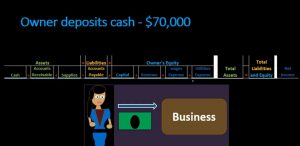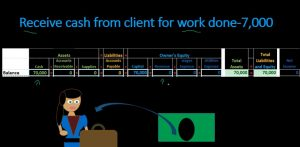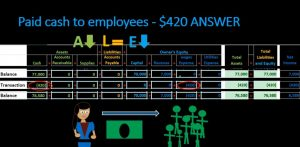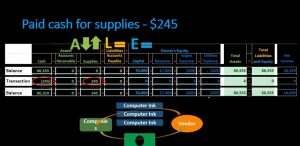# Cash Transaction – Accounting Equation 165

Hello, in this presentation we will be taking a look at business transactions involving cash we will be recording these normal business transactions in the format of the accounting equation and later be using the same or similar transactions to record with regard to debits and credits. Objectives. At the end of this we will be able to list transactions involving cash record transactions involving cash using the accounting equation. first transaction, we’re going to list through these transactions and we’re going to record these transactions with the accounting equation, learning these accounting equations and these transactions using our normal rules and thought process. So remember that this is our accounting equation, we’re going to have assets liabilities and equity.

00:49

When we record transactions using the accounting equation, we often use the accounts involved and this is sometimes called an extended accounting equation, a format that can be used To record transactions, it’s useful to start recording transactions in this format. And then later on move to debits and credits this format being useful because it is an equation, and therefore it’s going to be a good place to start because everybody has some experience with equations. However, as you can see just from this example, it does get a bit complex or a bit messy, and it’s much easier with debits and credits. And that’s the reason we will move to debits and credits. But the normal rules that will be the same when recording transactions or some rules will be the same. those rules will include that every transaction will have at least two accounts involved and every transaction will have an equal will remain in balance here meaning assets equal liabilities plus equity will always remain in balance.01:50

In this case, in the asset accounts, we have cash receivables and supplies. Under the liabilities accounts. We just have the accounts payable and in the owner capital, we add The capital and the entire Income Statement of revenue and expenses will then have the total assets equal in total liabilities and equity and see the impact on net income. first transaction owner deposits cash 70,001st question, I’m going to go through this thought process here. And we’ll continue with this process all the way through recording journal entries is cash affected. And in this case, we’re going to say, of course it is we’re dealing with the cash accounts. And therefore we’re going to be dealing with cash and cash is going to be increasing. So we’re going to increase cash and of course cash is within the assets section, then we need to know what the other side of the transaction is.

02:42

Now when we’re dealing with the owner depositing money, it would make sense for us to think of the owner’s equity or the owners capital account. If we’re talking about a corporation, it would be the selling of stock, and so and that would be stockholders, our capital, that would increase and this case is going to be the case capital account. So we have the 70,000. Within the capital account, we know that it’s increasing a couple different ways. One, we know that cash went up, and when cash goes up, if the other account is on the other side of the equal sign, it too, then must be going up. We also want to double check ourselves though, and see if it makes sense that capital would be going up bringing up the equity section. And we know that capital represents what is owed to the owner in a sense, meaning the company has assets at this point of 70,000.

03:32

And the company owes that either to a third party a liability or to the owner. In this case, it clearly owes that who has claimed to that 70,000 the owner not a third party, the owner is the one that put the money in the business. Therefore our debits equal our credits, we have the total debits here. Well, what am I talking the, the assets in our accounting equation are in balance in terms of assets equals liabilities plus x Equity meaning total assets equal total liabilities plus equity, no effect on net income. Next transaction, we’re going to start with this beginning balance. So this is going to be where we are starting at, then we’re going to add the new activity, the new activity being reflected here. And then we’ll get to the new balance. So note that as we record these transactions, we’re always going to start where we started in balance, we’re going to record something that will be in balance, and we will end with something that will remain in balance.

04:32

So next transaction, we’re going to say we received cash from client for work done 7000. So we received cash that’s going to be our first question is cash affected, key word is received. Therefore we know that cash is increasing. So we know that cash is affected, it is increasing. If cash goes up, then we need to know what other account is being affected here. Why are people paying us cash because we did work So when we do work we earn revenue. So here’s revenue, revenue is part of the equity section. And we can think of it in terms of if this side is going up assets going up, then this side must be going up, equity must be going up, and therefore revenue must be going up. Now when we consider the equity section, it can be a little bit confusing because the entire income statement including revenue and expenses are included in the equity section.05:28

So remember that net income is calculated as revenue minus expenses. If revenue goes up, net income goes up, whatever happens to net income will also be what is happening to total equity. When expenses go up, they bring net income down, which brings down equity. Therefore, if we take a look at our equation, we see that the cash is going up by the 7000 and the revenue is going up by the 7000. Note that revenue also only goes in one direction. If Only goes up as do all income statement accounts meaning revenue and expense accounts revenue and expense accounts only go in one direction, we can see now that our total assets equal our total liabilities plus equity as shown here. And that our net income has increased net income calculated as revenue of 7000 minus the expenses, which have as of this point, of course, there are none.

06:25

We then need the ending balance. So note that it’s not good enough to stop there, we need to say this beginning balance is in balance. This is what happened to this time. Where are we at? Where are we ending at, in this case, of course, cash is at 70,000. We got 7000 more, we are now at 77,000 in cash. Over here we had a capital account of 70,000. Nothing happened. So we’re just basically bringing that 70,000 down 70,000 plus zero. Here we have zero and then we have 7000 to increase and there’s our 7000 here It’s useful to bring all the other zeros down. So these other accounts, of course, nothing has happened, we’re just bringing those zeros down. And as we do that, then the total should remain in balance, meaning assets of 77,000 equal liabilities of 70,000 plus seven or 77,000 77,000 assets equals 77,000. Liabilities and Equity, and the total net income at 7000.

07:27

At this time, next transaction. Once again, we’re going to look at this beginning balance and note that we’re not looking at the beginning balance prior to the first beginning balance here. So in our prior slide, we’re eliminating this now now we’re really once we move on, we’re really just concerned with this part here. So that’s where we’re going to start with and then we’re going to do the same process. This is where we were before this transaction. This is going to be the transaction we record and then we’ll show where we are at after this transaction. So we have paid cash to employees, same questions we’re going to say is cash affected. key word here is paid, therefore, cash is going down. So we’re going to decrease cash this time. And then we just need to know what other account is affected.

08:13

Of course, cash is an asset. The other account that affected when we pay employees. Remember, we are not the employees here were the owner. So we are always recording these transactions in the as in the perspective of the business owner, and therefore we would have an expense of wages expense over here. Now we know it’s going down for a couple different reasons. Once we know what the wages expense, it might be a little bit more difficult for us to say, Hmm, wages expense going up or down. That’s why we focus on cash first, because if cash went down, and it’s on this side of the equal sign, then if this is the other side that something is happening to it then must be going down meaning it’s bringing down total equity. Now note that as we think about the expenses, if we double check it We might think that expenses only go one way expenses.

09:03

Typically, we typically think of expenses only going up. But when considering expenses, and as the relation to equity, they bring equity down. So keep that distinction in mind as we move to the next type of transactions I’ve we’ve as we move to debits and credits, we’re going to basically be saying that expenses go up. And that brings net income down and that brings down equity. So here we’re saying it goes down. And that doesn’t necessarily mean that expenses go down expenses, just go one way, bringing down the equity second section, bringing down net income. So if we were to record that, then we’re going to say cash is decreasing by the 420,000. And then that’s going to decrease the total assets of course, and then the equity is decreasing over here with the expense pulling down total equity.

09:56

And then we see that our total assets equal our total liability For this transaction, the 420 decrease in cash matching the 420 decrease in the equity due to expenses going up, we see that net income is affected by a net loss in this case, meaning revenue minus expenses are going down for this transaction, we have a loss of 420. Then we’re going to see our total here. So we have cash beginning balance of 77,000. This is what happened this time, we reduced cash by 420, leaving us with cash of 76 580. Then if we see what happened over here, in the last other side of the transaction we worked on, we had expenses of zero, we had more expenses, which means it’s going to bring down equity by 420. And we brought down that number, then we just need to bring down the numbers from the prior beginning balance before this transaction, including the capital of 70,000.10:54

Plus nothing happened this time means we have 70,000 revenue of 7000 Plus what happened this time gives us revenue of 7000. And we bring those down, the others are just bringing down the zeros, as you can see. So we have the zeros being brought down here, here, here and here. And then if we were to add up the total assets 76 580 cash being the only asset at this time would equal then the liabilities and equity of 70,000 plus 7000 minus 420,076 580. Total Assets equaling 76 580 total liabilities and equity net income at this time is now 6580, calculated as 7000 minus the 420. Next transaction, paid cash for supplies. So we’ll go through our normal thought process. Again, remember that I’m going to go back to the prior slide. This is the ending balance for this slide, which will then be the beginning balance for the next slide. We’re not worried about anything prior to that when Considering the new transaction, we’re just concerned with this balance, the new activity that will then happen, and then the new balance.

12:08

So we’ve got paid cash for supplies 240 is cash affected? Yes, it is. And then is it going up or down keyword paid, therefore, cash is going down. So we’re going to decrease cash, we’re gonna write that with arrows first decrease cash, then we need to see the other side of the transaction. Now, you might be thinking if we bought supplies, it should be some type of supplies expense. However, in the case of supplies, we typically are going to use supplies as our introduction to inventory meaning we’re going to put it on the books as an asset and then decrease it as we use it. And therefore we’re going to put it on the books as supplies an asset so we’re going to increase supplies the asset as it is consumed, we will decrease the asset asset and record the expense. So we got one asset Going up and one asset going down in this case, if we go look at this example here, then we see that cash is going down, we see that supplies is going up.

13:11

And this is going to be an unusual transaction in that we have one side of the equal sign both going down and going up. So that’s one an example of one of those just kind of unusual transactions that often throw people off. Because when asked what is the effect on the accounting equation, we’re typically wanting to say that cash is decreasing assets. And we have to remember that the others is increasing assets and therefore there’s no net effect on assets. And even though a transaction is happening, there’s no net effect on either assets, liabilities or equity, because the assets are going up and down. Nothing happened to liabilities, nothing happened to equity. Therefore, there’s no impact on total assets or total liabilities and equity from this transaction and no impact on net income. This one’s a In one reason that debits and credits are often a better tool than then using the accounting equation, because we will see a debit and a credit for each transaction, then we want to bring down the total.

14:12

So here’s our beginning balance. Here’s the transaction. Here’s the total. And note that as we look at this beginning balance, we do have an AI utilities expense that was recorded after the wages expense here. So if you’re looking at these numbers, and you’re saying it’s a difference, because we got the utilities expense 525 that was actually added another transaction added in between here. So then we’ve got this number, we’ve got the 86 555 minus the 245 gives us 286 310 in the cache, and then the supplies zero plus 245, gives us the 245. And then we need to bring down the rest of the numbers. 70 plus zero is 70,000 17,500 plus zero is two 17,500 negative 420 plus zero is negative 420 and 525 negative plus zero is 525.15:11

Negative, we know that the 86 310 plus the 245 for total assets equals the 86 555. And the total liabilities and equity of 70,000 plus 17,500 minus 420 minus 525 also equals 86,555. net income is at 16,555, calculated as revenue of 17,500 minus wages expenses of 420 minus utilities expenses of 525. And again, note that this last slide, we’re focusing in on the cache. So there are some transactions that happened after in between slides. Hear in that we have the revenue and the and the utilities and we’re focusing in here on the cash transaction related to the supplies. objectives, we are now able to list transaction transactions involving cash recorded transactions involving cash using the accounting equation.